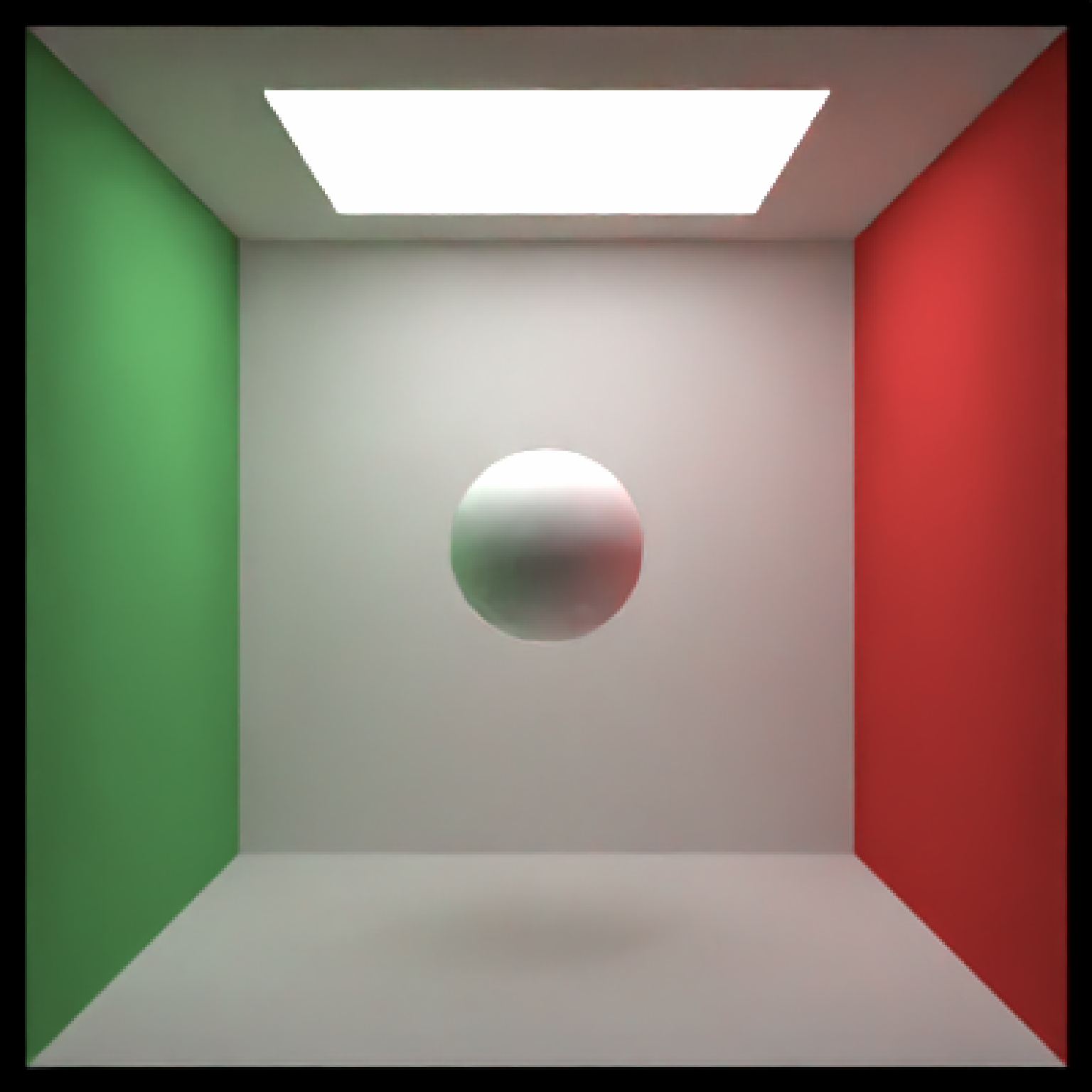Lambertian (diffuse) Material

lambertian(color = "#ffffff", checkercolor = NA, checkerperiod = 3,
noise = 0, noisephase = 0, noiseintensity = 10,
noisecolor = "#000000", image_array = NA, lightintensity = NA,
fog = FALSE, fogdensity = 0.01, implicit_sample = FALSE)

## Arguments

color Default white. The color of the surface. Can be either a hexadecimal code, R color string, or a numeric rgb vector listing three intensities between 0 and 1. Default NA. If not NA, determines the secondary color of the checkered surface. Can be either a hexadecimal code, or a numeric rgb vector listing three intensities between 0 and 1. Default 3. The period of the checker pattern. Increasing this value makes the checker pattern bigger, and decreasing it makes it smaller Default 0. If not 0, covers the surface in a turbulent marble pattern. This value will determine the amount of turbulence in the texture. Default 0. The phase of the noise. The noise will repeat at 360. Default 10. Intensity of the noise. Default #000000. The secondary color of the noise pattern. Can be either a hexadecimal code, or a numeric rgb vector listing three intensities between 0 and 1. A 3-layer RGB array to be used as the texture on the surface of the object. Default NA. If a positive value, this will turn this object into a light emitting the value specified in color (ignoring other properties). Higher values will produce a brighter light. Default FALSE. If TRUE, the object will be a volumetric scatterer. Default 0.01. The density of the fog. Higher values will produce more opaque objects. Default FALSE, unless the object is a light. If TRUE, the object will be sampled as part of the scattering probability density function.

## Value

Single row of a tibble describing the sphere in the scene.

## Examples

#Generate the cornell box and add a single white sphere to the center
scene = generate_cornell() %>%
aperture=0, fov=40, ambient_light=FALSE, parallel=TRUE)#Add a checkered rectangular cube below
scene = scene %>%
aperture=0, fov=40, ambient_light=FALSE, parallel=TRUE)aperture=0, fov=40, ambient_light=FALSE, parallel=TRUE)aperture=0, fov=40, ambient_light=FALSE, parallel=TRUE)